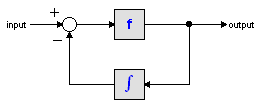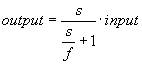﻿ 20-sim webhelp > Library > Iconic Diagrams > Mechanical > Translation > 2DSmallRotation > twodaccelerationsensor

# twodaccelerationsensor

Navigation:  Library > Iconic Diagrams > Mechanical > Translation > 2DSmallRotation >

# twodaccelerationsensor## Library

Iconic Diagrams\Mechanical\Translation\2DSmallAngles

## Use

Domains: Continuous. Size: 1-D/2-D. Kind: Iconic Diagrams (1D Translation, 1D Rotation, 2D Planar).

## Description

This model is used to find the absolute acceleration of a TwoDBody model. It has a port P which can be connected to the body and it has three output signals that denotes the x- and y-acceleration and the angular acceleration of the body.

The acceleration output is calculated out of the velocity by differentiation. Differentiation is performed by a state variable filter:The S-domain function of this filter is equal to:where f is the cut-off frequency. For very high values of f, the output becomes the pure derivative of the input. High values of f, however, increase simulations times. A good trade-off is a starting value of 1e5.

The equations of this model are:

P.F = 0;

ax = 2*pi*f*( P.v - int(ax, 0));

ay = 2*pi*f*( P.v - int(ay, 0));

alpha = 2*pi*f*( P.v - int(alpha, 0));

## Interface

 Ports Description P Port with three degrees of freedom (x, y, q). Causality fixed force out P Outputs ax Absolute acceleration [m]. ay Absolute acceleration [m]. alpha Absolute acceleration [rad]. Initial Values ax_initial Initial acceleration [m] ay_initial Initial acceleration [m] alpha_initial Initial angular acceleration [m]